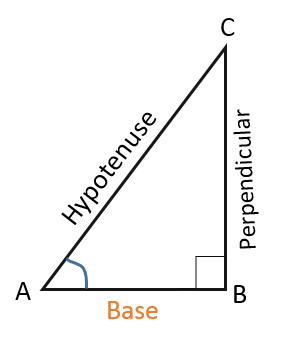# Basics of Trigonometry

As we already know about lines and angles, also their specific measurements in their respective units. Again we came to know about several geometrical figures such as triangle, Quadrilateral and polygons etc. only in a plane, say 2- dimension. All these studies of basics of geometry has only a single motive to find measurement of several aspects.

The word “Trigonometry is derived from Greek word “Tri” meaning three, “gon” meaning sides and “metron” meaning measurement.

For studying trigonometry, we have to be familiar with every property of triangle. Trigonometry mainly deals with the simple planar right-angled triangle.

A question must arise in your mind that “if trigonometry only deals with right triangle than what for triangles which are not right triangle?”

It is simple to answer as “Every triangle can be divided into two right triangles”. Except for right triangle as it is itself a right triangle (jokes apart). Most of the problems with these triangles are solved by reducing them to right triangles.

At this stage we are only studying the basics of trigonometry which is only in two dimensions, thus is planer. But Astronomers and space researchers study Spherical trigonometry which deals with trigonometry considering in a spherical body.

## Little Amazing History of Trigonometry:

• Have you ever read Vedas or Ramayana?  If you have than you must be aware of the “Hawan Kund” where there is given 36 + triangles around the Kund and is arranged symmetrically. This is one such example there are many such examples which states that Trigonometry has been first put in application by Indians.This is the start of basics of trigonometry.• Now, Let’s talk about the Pyramids of Europe. Of course, you have never visited there but you must know about it that its faces are triangular. Just think about its measurement that how such a large monument’s length had been premeasured before making it. Every face has same dimensions. All this leads to using trigonometric applications. This is the start of basics of trigonometry.## Terminology for Basics of Trigonometry:

Right Triangle: A triangle in which one of the angles has a measure of 90 and rest are acute angles is said as right triangle.“Remember: The two acute angle in a right triangle is complementary.”

Hypotenuse: It is the side opposite to right angle (90⁰). It is also the longest side of the triangle. Symbol to represent hypotenuse is “H”.

Base: The side adjacent to the acute angle which you are talking about is base. Symbol for base is “B”.

Perpendicular: The side opposite to the acute angle you are talking is called perpendicular. Symol to represent perpendicular is “P”.

## Trigonometric functions:

There are 6 different trigonometric functions:

1. Sine of x, written as sin(x)
2. Cosine of x, written as cos(x)
3. Tangent of x, written as Tan(x)
4. Cotangent of x, written as Cot(x)
5. Secant of x, written as Sec(x)
6. Cosecant of x, Written as Cosec(x)

## Trigonometric Ratios:

There are three primary trigonometric ratios. They are as follows:

Sine of ∠A = $$\frac{Perpendicular}{Hypotenuse}$$ = $$\frac{P}{H}$$

Cosine of ∠A = $$\frac{Base}{Hypotenuse}$$ = $$\frac{B}{H}$$

Tangent of ∠A = $$\frac{Perpendicular}{Base}$$ = $$\frac{P}{B}$$

And the rest are reciprocal of the above:

Cotangent of ∠A = $$\frac{1}{Tangent \:of \:∠A}$$ = $$\frac{Base}{Perpendicular}$$ = $$\frac{B}{P}$$

Secant of ∠A = $$\frac{1}{Cosine\: of\: ∠A}$$ = $$\frac{Hypotenuse}{Base}$$ = $$\frac{H}{B }$$

Cosecant of ∠A = $$\frac{1}{Sine\: of\: ∠A}$$ = $$\frac{Hypotenuse}{Perpendicular}$$ = $$\frac{H}{P}$$

## Table to learn trigonometric ratio for some specific angles.

 ∠A 0⁰ 30⁰ 45⁰ 60⁰ 90⁰ sin A 0 $$\frac{1}{2}$$ $$\frac{1}{\sqrt{2}}$$ $$\frac{\sqrt{3}}{2}$$ 1 cos A 1 $$\frac{\sqrt{3}}{2}$$ $$\frac{1}{\sqrt{2}}$$ $$\frac{1}{2}$$ 0 tan A 0 $$\frac{1}{\sqrt{3}}$$ 1 $$\sqrt{3}$$ Not defined cosec A Not defined 2 $$\sqrt{2}$$ $$\frac{2}{\sqrt{3}}$$ 1 sec A 1 $$\frac{2}{\sqrt{3}}$$ $$\sqrt{2}$$ 2 Not defined cot A Not defined $$\sqrt{3}$$ 1 $$\frac{1}{\sqrt{3}}$$ 0